# Maharashtra Board 8th Class Maths Practice Set 10.1 Solutions Chapter 10 Division of Polynomials

Balbharti Maharashtra State Board Class 8 Maths Solutions covers the Practice Set 10.1 8th Std Maths Answers Solutions Chapter 10 Division of Polynomials.

## Practice Set 10.1 8th Std Maths Answers Chapter 10 Division of Polynomials

Question 1.
Divide and write the quotient and the remainder.
i. 21m² ÷ 7m
ii. 40a³ ÷ (-10a)
iii. (- 48p4) ÷ (- 9p2)
iv. 40m5 ÷ 30m3
v. (5x3 – 3x2) ÷ x²
vi. (8p3 – 4p2) ÷ 2p2
vii. (2y3 + 4y2 + 3 ) ÷ 2y2
viii. (21x4 – 14x2 + 7x) ÷ 7x3
ix. (6x5 – 4x4 + 8x3 + 2x2) ÷ 2x2
x. (25m4 – 15m3 + 10m + 8) ÷ 5m3
Solution:
i. 21m² ÷ 7m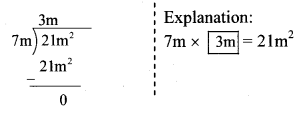∴ Quotient = 3m
Remainder = 0

ii. 40a³ ÷ (-10a)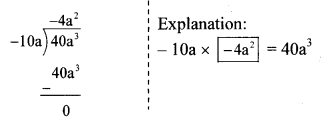∴ Quotient = -4a²
Remainder = 0

iii. (- 48p4) ÷ (- 9p2)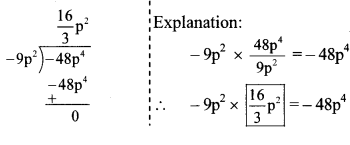∴ Quotient = $$\frac { 16 }{ 3 }$$ p²
Remainder = 0

iv. 40m5 ÷ 30m3∴ Quotient = $$\frac { 4 }{ 3 }$$ m²
Remainder = 0

v. (5x3 – 3x2) ÷ x²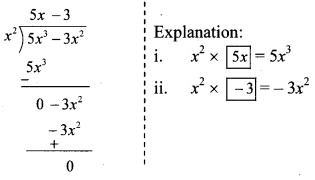∴ Quotient = 5x – 3
Remainder = 0

vi. (8p3 – 4p2) ÷ 2p2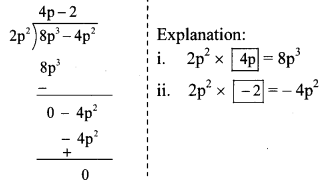∴ Quotient = 4p – 2
Remainder = 0

vii. (2y3 + 4y2 + 3 ) ÷ 2y2∴ Quotient = y + 2
Remainder = 3

viii. (21x4 – 14x2 + 7x) ÷ 7x3∴ Quotient = 3x
Remainder = -14x² + 7x

ix. (6x5 – 4x4 + 8x3 + 2x2) ÷ 2x2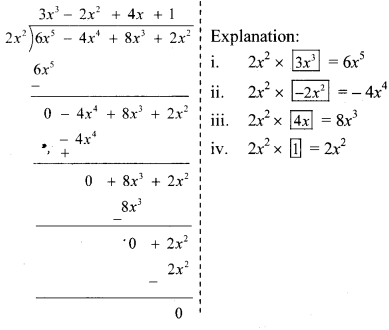∴ Quotient = 3x³ – 2x² + 4x + 1
Remainder = 0

x. (25m4 – 15m3 + 10m + 8) ÷ 5m3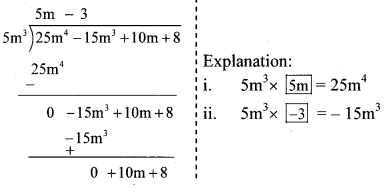∴ Quotient = 5m – 3
Remainder = 10m + 8

Maharashtra Board Class 8 Maths Chapter 10 Division of Polynomials Practice Set 10.1 Intext Questions and Activities

Question 1.
Fill in the blanks in the following examples. (Textbook pg. no. 61)

1. 2a + 3a = __
2. 7b – 4b = __
3. 3p × p² = __
4. 5m² × 3m² = __
5. (2x + 5y) × $$\frac { 3 }{ x }$$ = __
6. (3x² + 4y) × (2x + 3y) = __

Solution:

1. 2a + 3a = 5a
2. 7b – 4b = 3b
3. 3p × p² = 3p³
4. 5m² × 3m² = 15m4
5. (2x + 5y) × $$\frac { 3 }{ x }$$ = $$6+\frac { 15y }{ x }$$
6. (3x² + 4y) × (2x + 3y) = 6x³ + 9x²y + 8xy + 12y²
Scroll to Top